Want to share your content on R-bloggers? click here if you have a blog, or here if you don't.

MongoDB is a NoSQL database program which uses JSON-like documents with schemas. It is free and open-source cross-platform database. MongoDB, top NoSQL database engine in use today, could be a good data storage alternative when analyzing large volume data.
To use MongoDB with R, first, we have to download and install MongoDB
Next, start MongoDB. We can start MongoDB like so:

`mongod`

We can use the mongolite, package which is a fast and simple MongoDB client for R, to use MongoDB with R.

## Inserting data

Let’s insert the crimes data from data.gov to MongoDB. The dataset reflects reported incidents of crime (with the exception of murders where data exists for each victim) that occurred in the City of Chicago since 2001.

```library(ggplot2)
library(dplyr)
library(maps)
library(ggmap)
library(mongolite)
library(lubridate)
library(gridExtra)

names(crimes)
'ID' 'Case Number' 'Date' 'Block' 'IUCR' 'Primary Type' 'Description' 'Location Description' 'Arrest'
'Domestic' 'Beat' 'District' 'Ward' 'Community Area' 'FBI Code' 'X Coordinate' 'Y Coordinate' 'Year' 'Updated On' 'Latitude' 'Longitude' 'Location'```

Let’s remove spaces in the column names to avoid any problems when we query it from MongoDB.

```names(crimes) = gsub(" ","",names(crimes))
names(crimes)
'ID' 'CaseNumber' 'Date' 'Block' 'IUCR' 'PrimaryType' 'Description' 'LocationDescription' 'Arrest' 'Domestic' 'Beat' 'District' 'Ward' 'CommunityArea' 'FBICode' 'XCoordinate' 'YCoordinate' 'Year' 'UpdatedOn' 'Latitude' 'Longitude' 'Location'```

we can use the insert function from the mongolite package to insert rows to a collection in MongoDB.
Let’s create a database called Chicago and call the collection crimes.

```my_collection = mongo(collection = "crimes", db = "Chicago") # create connection, database and collection
my_collection\$insert(crimes)
```

Let’s check if we have inserted the “crimes” data.

```my_collection\$count()
6261148 ```

We see that the collection has 6261148 records.

## Performing a query and retrieving data

First, let’s look what the data looks like by displaying one record:

```my_collection\$iterate()\$one()
\$ID
1454164
\$CaseNumber
'G185744'
\$Date
'04/01/2001 06:00:00 PM'
\$Block
'049XX N MENARD AV'
\$IUCR
'0910'
\$PrimaryType
'MOTOR VEHICLE THEFT'
\$Description
'AUTOMOBILE'
\$LocationDescription
'STREET'
\$Arrest
'false'
\$Domestic
'false'
\$Beat
1622
\$District
16
\$FBICode
'07'
\$XCoordinate
1136545
\$YCoordinate
1932203
\$Year
2001
\$UpdatedOn
'08/17/2015 03:03:40 PM'
\$Latitude
41.970129962
\$Longitude
-87.773302309
\$Location
'(41.970129962, -87.773302309)'
```

How many distinct “Primary Type” do we have?

```length(my_collection\$distinct("PrimaryType"))
35```

As shown above, there are 35 different crime primary types in the database. We will see the patterns of the most common crime types below.
From the iterate()iterate() \$one() command above, we have seen that one of the columns is Domestic, which shows whether the crime is domestic or not.

### Now, let’s see how many domestic assualts there are in the collection.

```my_collection\$count('{"PrimaryType" : "ASSAULT", "Domestic" : "true" }')
82470

```

To get the filtered data and we can also retrieve only the columns of interest

```query1= my_collection\$find('{"PrimaryType" : "ASSAULT", "Domestic" : "true" }')
query2= my_collection\$find('{"PrimaryType" : "ASSAULT", "Domestic" : "true" }',
fields = '{"_id":0, "PrimaryType":1, "Domestic":1}')
ncol(query1) # with all the columns
ncol(query2) # only the selected columns
22
2
```

## Where do most crimes take pace?

```my_collection\$aggregate('[{"\$group":{"_id":"\$LocationDescription", "Count": {"\$sum":1}}}]')%>%na.omit()%>%
ggplot(aes(x=reorder(`_id`,Count),y=Count))+
geom_bar(stat="identity",color='skyblue',fill='#b35900')+geom_text(aes(label = Count), color = "blue") +coord_flip()+xlab("Location Description")
```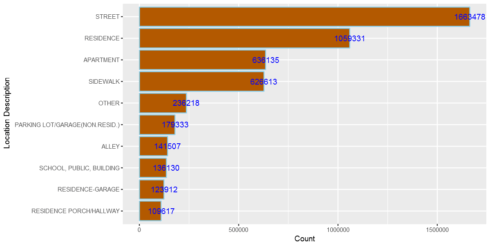If loading the entire dataset we are working with does not slow down our analysis, we can use data.table or dplyr but when dealing with big data, using MongoDB can give us performance boost as the whole data will not be loaded into mememory. We can reproduce the above plot without using MongoDB, like so:

```crimes%>%group_by(`LocationDescription`)%>%summarise(Total=n())%>% arrange(desc(Total))%>%head(10)%>%
ggplot(aes(x=reorder(`LocationDescription`,Total),y=Total))+
geom_bar(stat="identity",color='skyblue',fill='#b35900')+geom_text(aes(label = Total), color = "blue") +coord_flip()+xlab("Location Description")```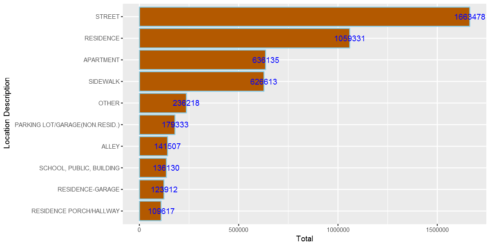What if we want to query all records for certain columns only? This helps us to load only the columns we want and to save memory for our analysis.

```query3= my_collection\$find('{}', fields = '{"_id":0, "Latitude":1, "Longitude":1,"Year":1}')
```

### Let’s explore domestic crimes

We can explore any patterns of domestic crimes. For example, are they common in certain days/hours/months?

```domestic=my_collection\$find('{"Domestic":"true"}', fields = '{"_id":0, "Domestic":1,"Date":1}')
domestic\$Date= mdy_hms(domestic\$Date)
domestic\$Weekday = weekdays(domestic\$Date)
domestic\$Hour = hour(domestic\$Date)
domestic\$month = month(domestic\$Date,label=TRUE)

WeekdayCounts = as.data.frame(table(domestic\$Weekday))
WeekdayCounts\$Var1 = factor(WeekdayCounts\$Var1, ordered=TRUE, levels=c("Sunday", "Monday", "Tuesday", "Wednesday", "Thursday", "Friday","Saturday"))
ggplot(WeekdayCounts, aes(x=Var1, y=Freq)) + geom_line(aes(group=1),size=2,color="red") + xlab("Day of the Week") + ylab("Total Domestic Crimes")+
ggtitle("Domestic Crimes in the City of Chicago Since 2001")+
theme(axis.title.x=element_blank(),axis.text.y = element_text(color="blue",size=11,angle=0,hjust=1,vjust=0),
axis.text.x = element_text(color="blue",size=11,angle=0,hjust=.5,vjust=.5),
axis.title.y = element_text(size=14),
plot.title=element_text(size=16,color="purple",hjust=0.5))
```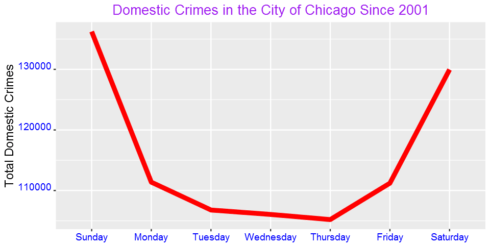Domestic crimes are common over the weekend than in weekdays? What could be the reason?
We can also see the pattern for each day by hour:

```DayHourCounts = as.data.frame(table(domestic\$Weekday, domestic\$Hour))
DayHourCounts\$Hour = as.numeric(as.character(DayHourCounts\$Var2))
ggplot(DayHourCounts, aes(x=Hour, y=Freq)) + geom_line(aes(group=Var1, color=Var1), size=1.4)+ylab("Count")+
ylab("Total Domestic Crimes")+ggtitle("Domestic Crimes in the City of Chicago Since 2001")+
theme(axis.title.x=element_text(size=14),axis.text.y = element_text(color="blue",size=11,angle=0,hjust=1,vjust=0),
axis.text.x = element_text(color="blue",size=11,angle=0,hjust=.5,vjust=.5),
axis.title.y = element_text(size=14),
legend.title=element_blank(),
plot.title=element_text(size=16,color="purple",hjust=0.5))
```The crimes peak mainly around mid-night. We can also use one color for weekdays and another color for weekend as shown below.

```DayHourCounts\$Type = ifelse((DayHourCounts\$Var1 == "Sunday") | (DayHourCounts\$Var1 == "Saturday"), "Weekend", "Weekday")

ggplot(DayHourCounts, aes(x=Hour, y=Freq)) + geom_line(aes(group=Var1, color=Type), size=2, alpha=0.5) +
ylab("Total Domestic Crimes")+ggtitle("Domestic Crimes in the City of Chicago Since 2001")+
theme(axis.title.x=element_text(size=14),axis.text.y = element_text(color="blue",size=11,angle=0,hjust=1,vjust=0),
axis.text.x = element_text(color="blue",size=11,angle=0,hjust=.5,vjust=.5),
axis.title.y = element_text(size=14),
legend.title=element_blank(),
plot.title=element_text(size=16,color="purple",hjust=0.5))
```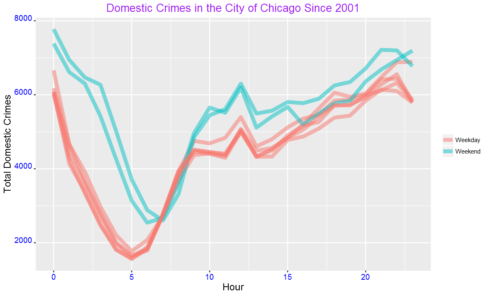The difference between weekend and weekdays are more clear from this figure than from the previous plot. We can also see the above pattern from a heatmap.

```DayHourCounts\$Var1 = factor(DayHourCounts\$Var1, ordered=TRUE, levels=c("Monday", "Tuesday", "Wednesday", "Thursday", "Friday", "Saturday", "Sunday"))
ggplot(DayHourCounts, aes(x = Hour, y = Var1)) + geom_tile(aes(fill = Freq)) + scale_fill_gradient(name="Total MV Thefts", low="white", high="red") +
ggtitle("Domestic Crimes in the City of Chicago Since 2001")+theme(axis.title.y = element_blank())+ylab("")+
theme(axis.title.x=element_text(size=14),axis.text.y = element_text(size=13),
axis.text.x = element_text(size=13),
axis.title.y = element_text(size=14),
legend.title=element_blank(),
plot.title=element_text(size=16,color="purple",hjust=0.5))
```From the heatmap, we can see more crimes over weekends and at night.

```monthCounts = as.data.frame(table(domestic\$month))
ggplot(monthCounts, aes(x=Var1, y=Freq)) + geom_line(aes(group=1),size=2,color="red") + xlab("Day of the Week") + ylab("Total Domestic Crimes")+
ggtitle("Domestic Crimes in the City of Chicago Since 2001")+
theme(axis.title.x=element_blank(),axis.text.y = element_text(color="blue",size=11,angle=0,hjust=1,vjust=0),
axis.text.x = element_text(color="blue",size=11,angle=0,hjust=.5,vjust=.5),
axis.title.y = element_text(size=14),
plot.title=element_text(size=16,color="purple",hjust=0.5))
```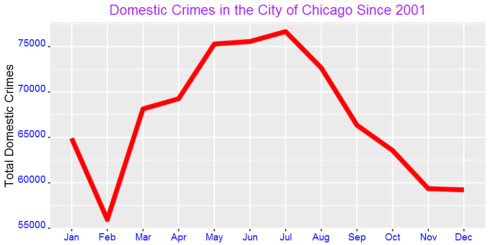Is domestic crime associated with temperature? Domestic crimes tremendously increases during the warm months.

Now, let’s see the pattern of other crime types. Since there are 35 primary types, we cannot see all of them in this post. Let’s focus on four of the most common ones.

```crimes=my_collection\$find('{}', fields = '{"_id":0, "PrimaryType":1,"Year":1}')
Imported 6261148 records. Simplifying into dataframe...
PrimaryType	Count
THEFT	        1301434
BATTERY	        1142377
CRIMINAL DAMAGE	720143
NARCOTICS	687790

```

As shown in the table above, the most common crime type is theft followed by battery. Narcotics is fourth most common while criminal damage is the third most common crime type in the city of Chicago.
Now, let’s generate plots by day and hour.

```four_most_common=crimes%>%group_by(PrimaryType)%>%summarize(Count=n())%>%arrange(desc(Count))%>%head(4)
four_most_common=four_most_common\$PrimaryType

crimes=my_collection\$find('{}', fields = '{"_id":0, "PrimaryType":1,"Date":1}')
crimes=filter(crimes,PrimaryType %in%four_most_common)
crimes\$Date= mdy_hms(crimes\$Date)
crimes\$Weekday = weekdays(crimes\$Date)
crimes\$Hour = hour(crimes\$Date)
crimes\$month=month(crimes\$Date,label = TRUE)

g = function(data){
WeekdayCounts = as.data.frame(table(data\$Weekday))
WeekdayCounts\$Var1 = factor(WeekdayCounts\$Var1, ordered=TRUE, levels=c("Sunday", "Monday", "Tuesday", "Wednesday", "Thursday", "Friday","Saturday"))
ggplot(WeekdayCounts, aes(x=Var1, y=Freq)) + geom_line(aes(group=1),size=2,color="red") + xlab("Day of the Week") +
theme(axis.title.x=element_blank(),axis.text.y = element_text(color="blue",size=10,angle=0,hjust=1,vjust=0),
axis.text.x = element_text(color="blue",size=10,angle=0,hjust=.5,vjust=.5),
axis.title.y = element_text(size=11),
plot.title=element_text(size=12,color="purple",hjust=0.5))
}

g1=g(filter(crimes,PrimaryType=="THEFT"))+ggtitle("Theft")+ylab("Total Count")
g2=g(filter(crimes,PrimaryType=="BATTERY"))+ggtitle("BATTERY")+ylab("Total Count")
g3=g(filter(crimes,PrimaryType=="CRIMINAL DAMAGE"))+ggtitle("CRIMINAL DAMAGE")+ylab("Total Count")
g4=g(filter(crimes,PrimaryType=="NARCOTICS"))+ggtitle("NARCOTICS")+ylab("Total Count")
grid.arrange(g1,g2,g3,g4,ncol=2)
```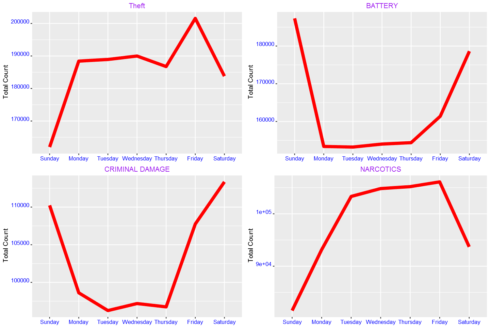From the plots above, we see that theft is most common on Friday. Battery and criminal damage, on the other hand, are highest at weekend. We also observe that narcotics decreases over weekend.
We can also see the pattern of the above four crime types by hour:

```g=function(data){
DayHourCounts = as.data.frame(table(data\$Weekday, data\$Hour))
DayHourCounts\$Hour = as.numeric(as.character(DayHourCounts\$Var2))
ggplot(DayHourCounts, aes(x=Hour, y=Freq)) + geom_line(aes(group=Var1, color=Var1), size=1.4)+ylab("Count")+
theme(axis.title.x=element_text(size=14),axis.text.y = element_text(color="blue",size=11,angle=0,hjust=1,vjust=0),
axis.text.x = element_text(color="blue",size=11,angle=0,hjust=.5,vjust=.5),
axis.title.y = element_text(size=14),
legend.title=element_blank(),
plot.title=element_text(size=16,color="purple",hjust=0.5))
}

g1=g(filter(crimes,PrimaryType=="THEFT"))+ggtitle("Theft")+ylab("Total Count")
g2=g(filter(crimes,PrimaryType=="BATTERY"))+ggtitle("BATTERY")+ylab("Total Count")
g3=g(filter(crimes,PrimaryType=="CRIMINAL DAMAGE"))+ggtitle("CRIMINAL DAMAGE")+ylab("Total Count")
g4=g(filter(crimes,PrimaryType=="NARCOTICS"))+ggtitle("NARCOTICS")+ylab("Total Count")
grid.arrange(g1,g2,g3,g4,ncol=2)
```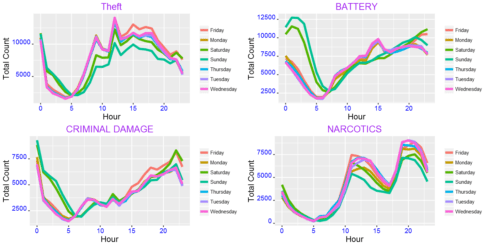```g=function(data){
monthCounts = as.data.frame(table(data\$month))
ggplot(monthCounts, aes(x=Var1, y=Freq)) + geom_line(aes(group=1),size=2,color="red") + xlab("Day of the Week") +
theme(axis.title.x=element_blank(),axis.text.y = element_text(color="blue",size=11,angle=0,hjust=1,vjust=0),
axis.text.x = element_text(color="blue",size=11,angle=0,hjust=.5,vjust=.5),
axis.title.y = element_text(size=14),
plot.title=element_text(size=16,color="purple",hjust=0.5))
}

g1=g(filter(crimes,PrimaryType=="THEFT"))+ggtitle("Theft")+ylab("Total Count")
g2=g(filter(crimes,PrimaryType=="BATTERY"))+ggtitle("BATTERY")+ylab("Total Count")
g3=g(filter(crimes,PrimaryType=="CRIMINAL DAMAGE"))+ggtitle("CRIMINAL DAMAGE")+ylab("Total Count")
g4=g(filter(crimes,PrimaryType=="NARCOTICS"))+ggtitle("NARCOTICS")+ylab("Total Count")
grid.arrange(g1,g2,g3,g4,ncol=2)
```Except, narcotics, all increase in the warmer months. Does this have any association with temperature?

### We can also produce maps.

```chicago = get_map(location = "chicago", zoom = 11) # Load a map of Chicago into R:
```

Round our latitude and longitude to 2 digits of accuracy, and create a crime counts data frame for each area:

```query3= my_collection\$find('{}', fields = '{"_id":0, "Latitude":1, "Longitude":1,"Year":1}')
LatLonCounts=as.data.frame(table(round(query3\$Longitude,2),round(query3\$Latitude,2)))
```

Convert our Longitude and Latitude variable to numbers:

```LatLonCounts\$Long = as.numeric(as.character(LatLonCounts\$Var1))
LatLonCounts\$Lat = as.numeric(as.character(LatLonCounts\$Var2))
ggmap(chicago) + geom_tile(data = LatLonCounts, aes(x = Long, y = Lat, alpha = Freq), fill="red")+
ggtitle("Crime Distribution")+labs(alpha="Count")+theme(plot.title = element_text(hjust=0.5))
```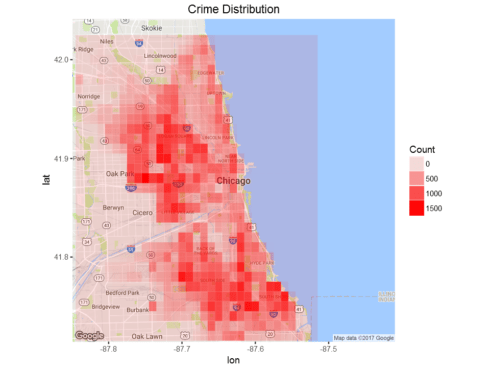We can also see a map for domestic crimes only:

```domestic=my_collection\$find('{"Domestic":"true"}', fields = '{"_id":0, "Latitude":1, "Longitude":1,"Year":1}')
LatLonCounts=as.data.frame(table(round(domestic\$Longitude,2),round(domestic\$Latitude,2)))
LatLonCounts\$Long = as.numeric(as.character(LatLonCounts\$Var1))
LatLonCounts\$Lat = as.numeric(as.character(LatLonCounts\$Var2))
ggmap(chicago) + geom_tile(data = LatLonCounts, aes(x = Long, y = Lat, alpha = Freq), fill="red")+
ggtitle("Domestic Crimes")+labs(alpha="Count")+theme(plot.title = element_text(hjust=0.5))
```
```mtheft=my_collection\$find('{"PrimaryType":"MOTOR VEHICLE THEFT"}', fields = '{"_id":0, "Latitude":1, "Longitude":1,"Year":1}')
LatLonCounts=as.data.frame(table(round(mtheft\$Longitude,2),round(mtheft\$Latitude,2)))
LatLonCounts\$Long = as.numeric(as.character(LatLonCounts\$Var1))
LatLonCounts\$Lat = as.numeric(as.character(LatLonCounts\$Var2))
ggmap(chicago) + geom_tile(data = LatLonCounts, aes(x = Long, y = Lat, alpha = Freq), fill="red")+
ggtitle("Motor Vehicle Theft ")+labs(alpha="Count")+theme(plot.title = element_text(hjust=0.5))
```Domestic crimes show concentration over two areas whereas motor vehicle theft is wide spread over large part of the city of Chicago.

## Summary

In this post, we saw how to use R to analyse data stored in MongoDB, top NoSQL database engine in use today. When dealing with large volume data, using MongoDB can give us performance boost and make our life happierRelated Post